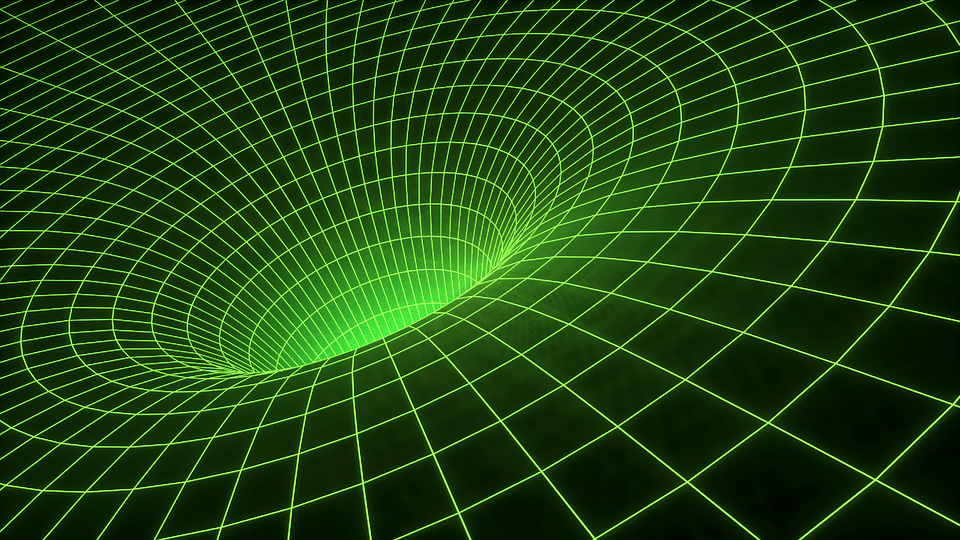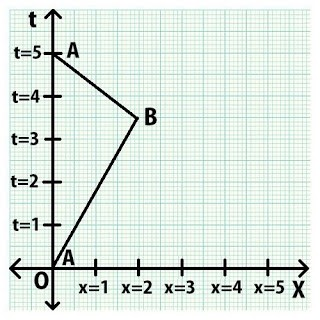To understand relativity you just need an understanding of basic algebra. However, things become truly nasty when we start thinking about the events in different frames of reference. One of the best solutions to visualize the transformation between the reference frames between any observers is Minkowski’s spacetime diagram. I hope you have read my last post, ‘Relativity for beginners‘ and are ready to go to the next level. In this post, I shall be taking you on a journey through relativity where you shall be learning about spacetime diagrams, light-cones, spacetime interval, and causality.

So let’s consider the graph 1, to calculate the distance between the two points we can simply apply distance formula:

D2 = (x2 – x1)2 + (y2 – y1)2graph 2 1. I going from point A to point B  2. I come to my original position.

Which basically comes from Pythagoras theorem. Now we simply replace our y-axis with time t and we get a graph similar to graph 2. In graph 2 we have plotted two events; one I going from point A to point B and two I coming to my original position i.e. point A. Do note that this is a linear i.e. one-dimensional motion but since we have added time as our second coordinate, we are plotting my events in two-dimensional spacetime.

Let’s consider graph 3 where the events happening are from my perspective and not from your/ third person’s perspective. For the simplicity of imagination let’s say I see a person riding on a horse. From my perspective I am stationary and the rider is moving with velocity ‘v’. But from the rider’s perspective/ reference frame (constant velocity/ no acceleration), he is stationary and I am moving. Therefore if I want to plot my spacetime diagram it would be like graph 3.1 and if the rider is plotting the graph, then, his spacetime diagram would be like graph 3.2.

In both the graphs, we observe that time and distance covered by him or me is the same as that of me or him. The graph basically defines the Newtonian understanding of space and time as two different entities and time and space to be absolute. However, in the relativity world, we know that time dilates and length contracts. Therefore the graph 3.1 and 3.2 are wrong.

In relativity space and time are two components of a single spacetime, where space and time are not absolute, it varies for different observers. ‘Transformations’ are what required to change from one reference frame to another reference frame. Such transformations were developed by Galileo Galilei and then upgraded by Lorentz. The problem with Galilean transformation was that space and time were independent of the velocity, whereas the Lorentz transformation showed the dependency of space and time on velocity by inserting the Lorentz factor. So when we change the reference frame from me to the rider the correct spacetime diagram for graph3.2 will be like graph4.2 and the ticks (mark) between the time and space intervals (U’) for the rider can be calculated by:

U’ = U [√(1+β2) ÷ √(1 – β2)]

Where,
U = unit length of x and ct axes
U’ = unit length of x’ and ct’ axes
β = v/c = tan(α) [will be discussed shortly]